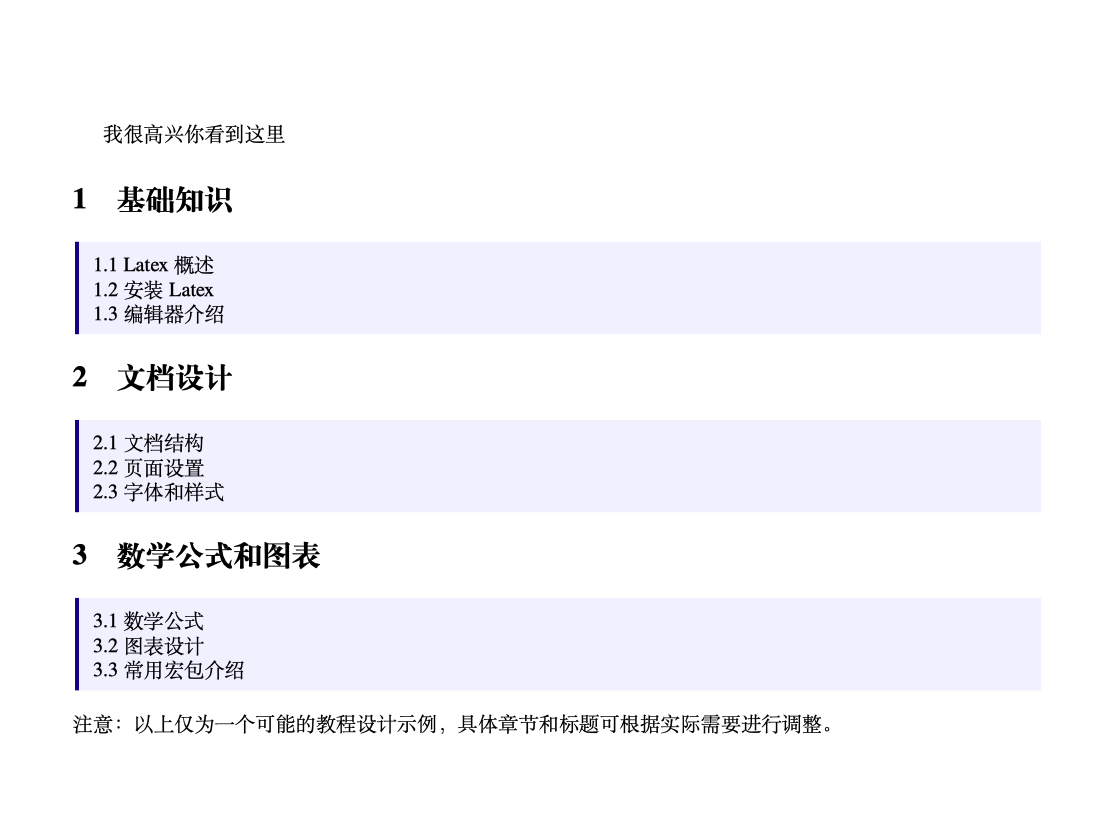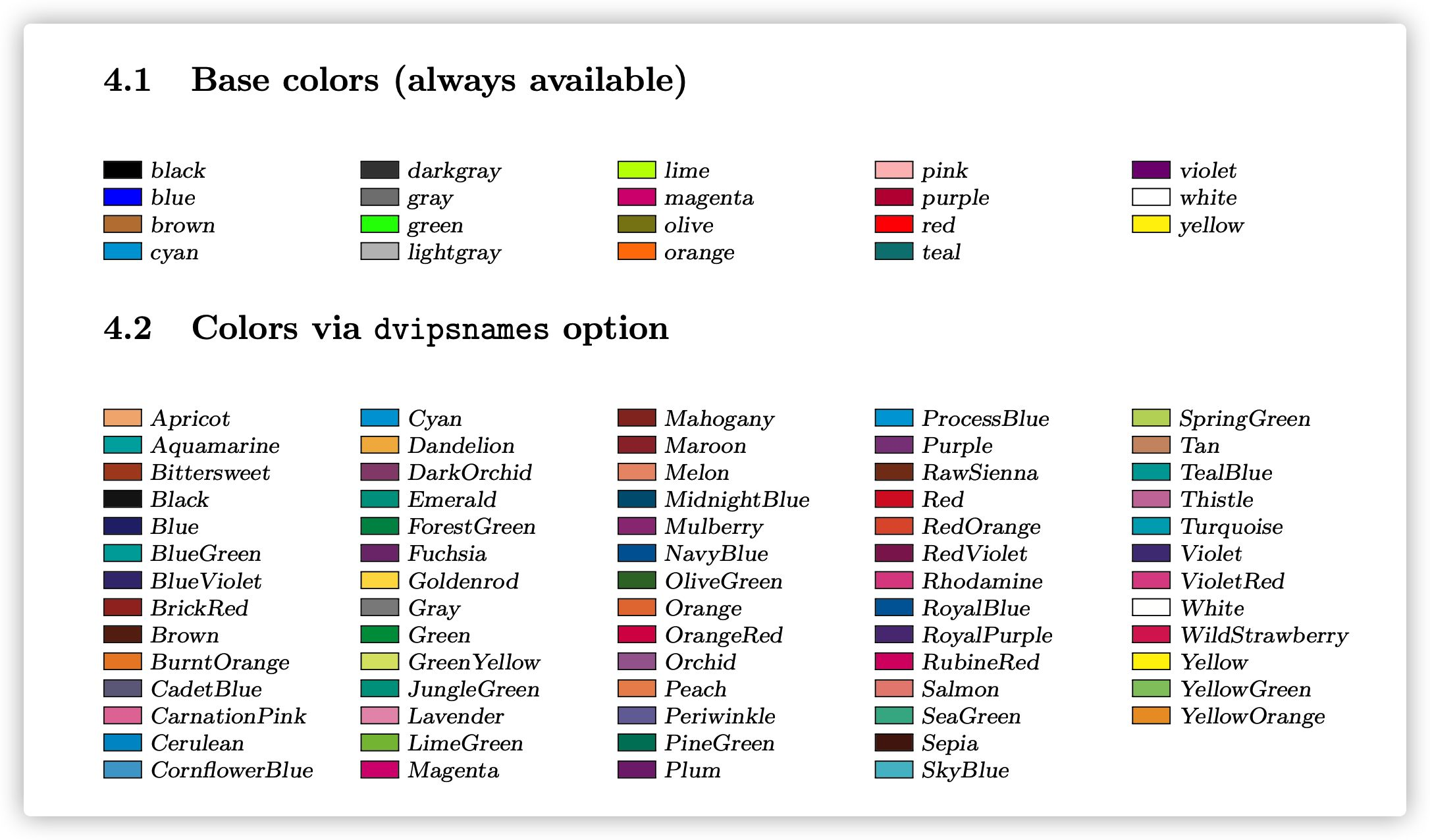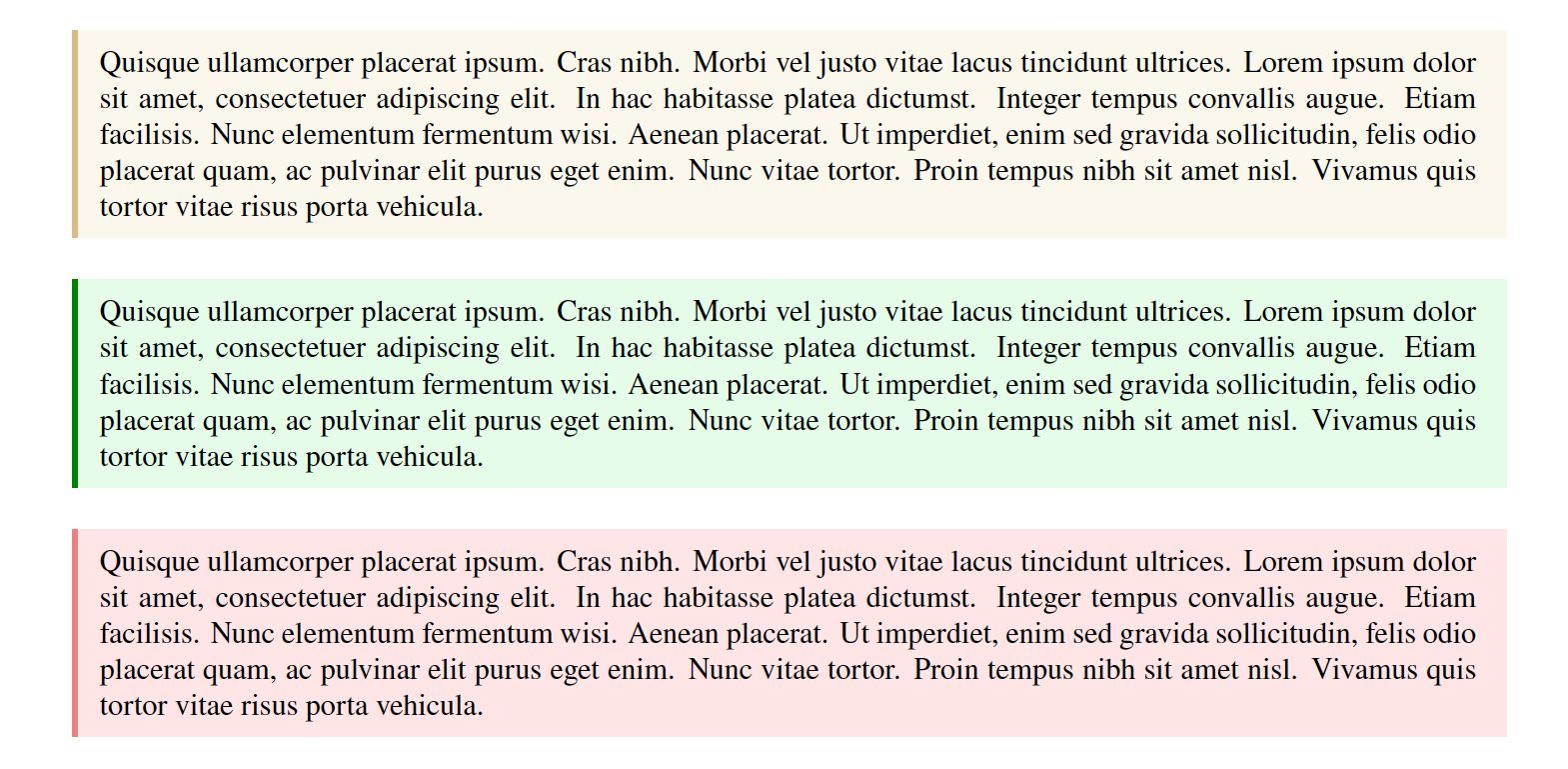## 一、渲染效果### 二、源码#

% 介绍文本框底纹填充效果，原作者：https://jevon.org/wiki/Fancy_Quotation_Boxes_in_Latex
\documentclass{article}
\usepackage{xeCJK}
\usepackage{newtxtext}
\usepackage{geometry}
\usepackage{lipsum} % 该宏包是通过 \lipsum 命令生成一段本文，正式使用时不需要引用该宏包
\usepackage[dvipsnames,svgnames]{xcolor}
\usepackage{framed} % 实现方框效果

\geometry{a4paper,centering,scale=0.8}
% environment derived from framed.sty: see leftbar environment definition
% ------------------******-------------------
% 注意行末需要把空格注释掉，不然画出来的方框会有空白竖线
\newenvironment{formal}{%
\def\FrameCommand{%
\hspace{1pt}%
{\color{DarkBlue}\vrule width 2pt}%
}%
\noindent\hspace{-4.55pt}% disable indenting first paragraph
\vspace{2pt}\vspace{2pt}%
}
{%
}
% ------------------******-------------------
\begin{document}

\section{基础知识}
\begin{formal}
1.1 Latex概述\\
1.2 安装Latex\\
1.3 编辑器介绍
\end{formal}

\section{文档设计}
\begin{formal}
2.1 文档结构\\
2.2 页面设置\\
2.3 字体和样式
\end{formal}

\section{数学公式和图表}
\begin{formal}
3.1 数学公式\\
3.2 图表设计\\
3.3 常用宏包介绍
\end{formal}

\end{document}


## 三、细节#

### 3.2 修改竖线颜色#\definecolor{greenshade}{rgb}{0.90,0.99,0.91} % 绿色文本框，竖线颜色设为 Green